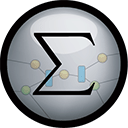# Top 20 NuGet numeric Packages

Math.NET Numerics is the numerical foundation of the Math.NET project, aiming to provide methods and algorithms for numerical computations in science, engineering and every day use. Supports .Net Framework 4.0 or higher and .Net Standard 1.3 or higher, on Windows, Linux and Mac.## Math.NET Numerics - Strong Name Edition

Math.NET Numerics is the numerical foundation of the Math.NET project, aiming to provide methods and algorithms for numerical computations in science, engineering and every day use. Supports .Net Framework 4.0 or higher and .Net Standard 1.3 or higher, on Windows, Linux and Mac. This package contain...
F# Modules for Math.NET Numerics, the numerical foundation of the Math.NET project, aiming to provide methods and algorithms for numerical computations in science, engineering and every day use. Supports .Net Framework 4.5 or higher and .Net Standard 1.6 or higher, on Windows, Linux and Mac.## Math.NET Numerics for F# - Strong Name Edition

F# Modules for Math.NET Numerics, the numerical foundation of the Math.NET project, aiming to provide methods and algorithms for numerical computations in science, engineering and every day use. Supports .Net Framework 4.5 or higher and .Net Standard 1.6 or higher, on Windows, Linux and Mac. This pa...
NumSharp is the fundamental library for scientific computing with .NET providing a similar API to python's numpy scientific library. NumSharp has full N-D, broadcasting and axis support. If you want to use .NET to get started with machine learning, NumSharp will be your best tool.
Syncfusion Input controls for Windows Forms provides various input controls needed in line of business applications. This package contains the following controls. Calendar: https://www.syncfusion.com/winforms-ui-controls/calendar?utm_source=nuget&utm_medium=listing Date-Time Edit: https://www...
Syncfusion input controls for UWP provide various input controls commonly used in line-of-business applications. This package contains the following controls. Calculator: https://www.syncfusion.com/uwp-ui-controls/calculator?utm_source=nuget&utm_medium=listing Calendar: https://www.syncfusion.co...
A library for advanced numerical computing, with support for data wrangling, statistics, matrix algebra, advanced functions, numerical integration and optimization, and Fourier transforms.
jQuery plugin that automatically formats currency (money) and numbers as you type on form inputs. It supports most International numeric formats and currency signs including those used in Europe, North and South America, Afirica, Asia and India lakhs
Provides a numeric up down WPF control that can be used to edit (integer, byte, decimal, double, float, short and many more) values with a textbox and/or up/down arrow (repeat) buttons. Editing can be based on input via mouse wheel, mouse drag, cursor keys, or text editing.## Math.NET Numerics - Text Data I/O Extensions

Text Data Input/Output Extensions for Math.NET Numerics, the numerical foundation of the Math.NET project, aiming to provide methods and algorithms for numerical computations in science, engineering and every day use.
DevExpress.XAF.Extensions.Numeric## Math.NET Numerics - MATLAB Data I/O Extensions

MathWorks MATLAB Data Input/Output Extensions for Math.NET Numerics, the numerical foundation of the Math.NET project, aiming to provide methods and algorithms for numerical computations in science, engineering and every day use.
Extended collection of packages that provide common structures, extensions, and helpers that can be used across many libraries, and applications.## Math.NET Numerics - MKL Native Provider for Windows (x64)

Intel MKL native libraries for Math.NET Numerics on Windows.## Math.NET Numerics - MKL Native Provider for Windows (x64 and x86)

Intel MKL native libraries for Math.NET Numerics on Windows.## Math.NET Numerics - OpenBLAS Native Provider for Windows (x64 and x86)

OpenBLAS native libraries for Math.NET Numerics.
Allows only valid characters (i.e. numbers) to be typed into a text box. Can take negative numbers and a decimal point. You can supply a callback that runs when focus is lost and the value in the text box is not a valid number.## Laavor TextBox Validation(Numeric, Email, Text) for Xamarin (Android, iOS, UWP)

The component contains a versatile and easy-to-use validation, giving dynamism to your application, has a form that takes the auto validate components and enables the auto submit button when the data is valid. They can also be used independently for numbers, string and email. You can receive a label...
.NET FRAMEWORK 4 A WPF TextBox for entering a Currency/Numeric/Percent value, similar to how a cash register works. Numbers typed are pushed in from the right.